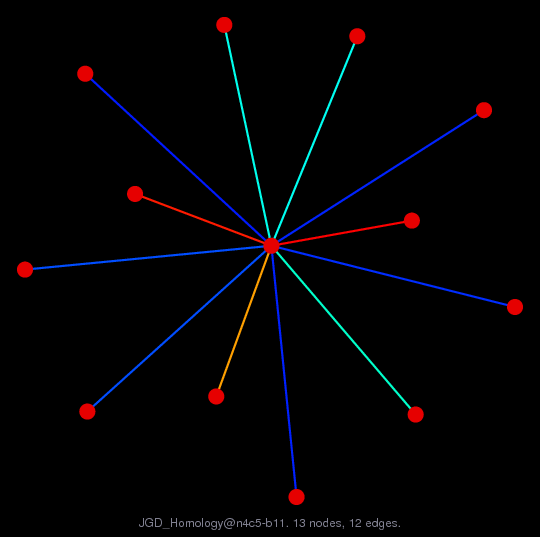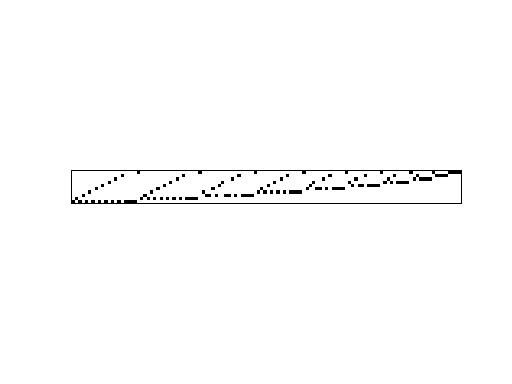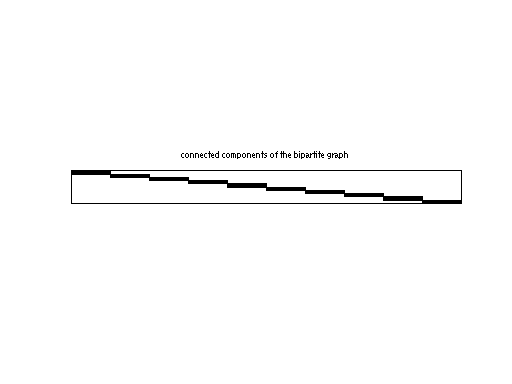Matrix: JGD_Homology/n4c5-b11

Description: Simplicial complexes from Homology from Volkmar Welker.(bipartite graph drawing)• Matrix group: JGD_Homology
• download as a MATLAB mat-file, file size: 1 KB. Use UFget(2109) or UFget('JGD_Homology/n4c5-b11') in MATLAB.

 Matrix properties number of rows 10 number of columns 120 nonzeros 120 structural full rank? yes structural rank 10 # of blocks from dmperm 1 # strongly connected comp. 10 explicit zero entries 0 nonzero pattern symmetry 0% numeric value symmetry 0% type integer structure rectangular Cholesky candidate? no positive definite? no

 author V. Welker editor J.-G. Dumas date 2008 kind combinatorial problem 2D/3D problem? no

Notes:

```Simplicial complexes from Homology from Volkmar Welker.
From Jean-Guillaume Dumas' Sparse Integer Matrix Collection,
http://ljk.imag.fr/membres/Jean-Guillaume.Dumas/simc.html

http://www.mathematik.uni-marburg.de/~welker/

Filename in JGD collection: Homology/n4c5.b11.10x120.sms
```

 Ordering statistics: result nnz(V) for QR, upper bound nnz(L) for LU, with COLAMD 120 nnz(R) for QR, upper bound nnz(U) for LU, with COLAMD 10

 SVD-based statistics: norm(A) 3.4641 min(svd(A)) 3.4641 cond(A) 1 rank(A) 10 sprank(A)-rank(A) 0 null space dimension 0 full numerical rank? yes

 singular values (MAT file): click here SVD method used: s = svd (full (R)) ; where [~,R,E] = spqr (A') with droptol of zero status: ok